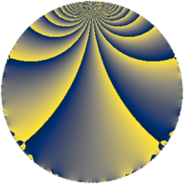Properties

 Label 8280.2.a.bkLevel $8280$ Weight $2$ Character orbit 8280.a Self dual yes Analytic conductor $66.116$ Analytic rank $1$ Dimension $3$ CM no Inner twists $1$

Related objects

Newspace parameters

 Level: $$N$$ $$=$$ $$8280 = 2^{3} \cdot 3^{2} \cdot 5 \cdot 23$$ Weight: $$k$$ $$=$$ $$2$$ Character orbit: $$[\chi]$$ $$=$$ 8280.a (trivial)

Newform invariants

 Self dual: yes Analytic conductor: $$66.1161328736$$ Analytic rank: $$1$$ Dimension: $$3$$ Coefficient field: 3.3.568.1 Defining polynomial: $$x^{3} - x^{2} - 6 x - 2$$ Coefficient ring: $$\Z[a_1, \ldots, a_{7}]$$ Coefficient ring index: $$1$$ Twist minimal: yes Fricke sign: $$1$$ Sato-Tate group: $\mathrm{SU}(2)$

$q$-expansion

Coefficients of the $$q$$-expansion are expressed in terms of a basis $$1,\beta_1,\beta_2$$ for the coefficient ring described below. We also show the integral $$q$$-expansion of the trace form.

 $$f(q)$$ $$=$$ $$q - q^{5} + ( 1 + \beta_{1} ) q^{7} +O(q^{10})$$ $$q - q^{5} + ( 1 + \beta_{1} ) q^{7} + ( -2 - \beta_{1} - \beta_{2} ) q^{11} + ( \beta_{1} + \beta_{2} ) q^{13} + ( -1 - \beta_{1} ) q^{17} + ( 2 - \beta_{1} + \beta_{2} ) q^{19} + q^{23} + q^{25} + ( 1 - \beta_{2} ) q^{29} + ( -1 - \beta_{2} ) q^{31} + ( -1 - \beta_{1} ) q^{35} + ( 1 - \beta_{1} ) q^{37} + ( -5 - \beta_{2} ) q^{41} + ( 2 \beta_{1} + 2 \beta_{2} ) q^{43} + ( -6 + \beta_{1} - \beta_{2} ) q^{47} + ( -2 + 4 \beta_{1} + \beta_{2} ) q^{49} + ( -1 - \beta_{1} ) q^{53} + ( 2 + \beta_{1} + \beta_{2} ) q^{55} + ( -1 + 2 \beta_{1} + 3 \beta_{2} ) q^{59} + ( 4 - \beta_{1} - 3 \beta_{2} ) q^{61} + ( -\beta_{1} - \beta_{2} ) q^{65} + ( 1 - \beta_{1} - 4 \beta_{2} ) q^{67} + ( -1 - 4 \beta_{1} + 3 \beta_{2} ) q^{71} + ( 4 - 5 \beta_{1} - 3 \beta_{2} ) q^{73} + ( -4 - 5 \beta_{1} - \beta_{2} ) q^{77} + 4 \beta_{2} q^{79} + ( -3 + \beta_{1} + 2 \beta_{2} ) q^{83} + ( 1 + \beta_{1} ) q^{85} + ( 8 - 2 \beta_{1} + 2 \beta_{2} ) q^{89} + ( 2 + 3 \beta_{1} + \beta_{2} ) q^{91} + ( -2 + \beta_{1} - \beta_{2} ) q^{95} + ( -2 - 2 \beta_{1} + 2 \beta_{2} ) q^{97} +O(q^{100})$$ $$\operatorname{Tr}(f)(q)$$ $$=$$ $$3 q - 3 q^{5} + 4 q^{7} + O(q^{10})$$ $$3 q - 3 q^{5} + 4 q^{7} - 6 q^{11} - 4 q^{17} + 4 q^{19} + 3 q^{23} + 3 q^{25} + 4 q^{29} - 2 q^{31} - 4 q^{35} + 2 q^{37} - 14 q^{41} - 16 q^{47} - 3 q^{49} - 4 q^{53} + 6 q^{55} - 4 q^{59} + 14 q^{61} + 6 q^{67} - 10 q^{71} + 10 q^{73} - 16 q^{77} - 4 q^{79} - 10 q^{83} + 4 q^{85} + 20 q^{89} + 8 q^{91} - 4 q^{95} - 10 q^{97} + O(q^{100})$$

Basis of coefficient ring in terms of a root $$\nu$$ of $$x^{3} - x^{2} - 6 x - 2$$:

 $$\beta_{0}$$ $$=$$ $$1$$ $$\beta_{1}$$ $$=$$ $$\nu$$ $$\beta_{2}$$ $$=$$ $$\nu^{2} - 2 \nu - 4$$
 $$1$$ $$=$$ $$\beta_0$$ $$\nu$$ $$=$$ $$\beta_{1}$$ $$\nu^{2}$$ $$=$$ $$\beta_{2} + 2 \beta_{1} + 4$$

Embeddings

For each embedding $$\iota_m$$ of the coefficient field, the values $$\iota_m(a_n)$$ are shown below.

For more information on an embedded modular form you can click on its label.

Label $$\iota_m(\nu)$$ $$a_{2}$$ $$a_{3}$$ $$a_{4}$$ $$a_{5}$$ $$a_{6}$$ $$a_{7}$$ $$a_{8}$$ $$a_{9}$$ $$a_{10}$$
1.1
 −1.76156 −0.363328 3.12489
0 0 0 −1.00000 0 −0.761557 0 0 0
1.2 0 0 0 −1.00000 0 0.636672 0 0 0
1.3 0 0 0 −1.00000 0 4.12489 0 0 0
 $$n$$: e.g. 2-40 or 990-1000 Significant digits: Format: Complex embeddings Normalized embeddings Satake parameters Satake angles

Atkin-Lehner signs

$$p$$ Sign
$$2$$ $$-1$$
$$3$$ $$1$$
$$5$$ $$1$$
$$23$$ $$-1$$

Inner twists

This newform does not admit any (nontrivial) inner twists.

Twists

By twisting character orbit
Char Parity Ord Mult Type Twist Min Dim
1.a even 1 1 trivial 8280.2.a.bk 3
3.b odd 2 1 8280.2.a.bp yes 3

By twisted newform orbit
Twist Min Dim Char Parity Ord Mult Type
8280.2.a.bk 3 1.a even 1 1 trivial
8280.2.a.bp yes 3 3.b odd 2 1

Hecke kernels

This newform subspace can be constructed as the intersection of the kernels of the following linear operators acting on $$S_{2}^{\mathrm{new}}(\Gamma_0(8280))$$:

 $$T_{7}^{3} - 4 T_{7}^{2} - T_{7} + 2$$ $$T_{11}^{3} + 6 T_{11}^{2} + 2 T_{11} - 20$$ $$T_{13}^{3} - 10 T_{13} + 8$$ $$T_{17}^{3} + 4 T_{17}^{2} - T_{17} - 2$$

Hecke characteristic polynomials

$p$ $F_p(T)$
$2$ $$T^{3}$$
$3$ $$T^{3}$$
$5$ $$( 1 + T )^{3}$$
$7$ $$2 - T - 4 T^{2} + T^{3}$$
$11$ $$-20 + 2 T + 6 T^{2} + T^{3}$$
$13$ $$8 - 10 T + T^{3}$$
$17$ $$-2 - T + 4 T^{2} + T^{3}$$
$19$ $$-8 - 14 T - 4 T^{2} + T^{3}$$
$23$ $$( -1 + T )^{3}$$
$29$ $$10 - 3 T - 4 T^{2} + T^{3}$$
$31$ $$-4 - 7 T + 2 T^{2} + T^{3}$$
$37$ $$8 - 5 T - 2 T^{2} + T^{3}$$
$41$ $$64 + 57 T + 14 T^{2} + T^{3}$$
$43$ $$64 - 40 T + T^{3}$$
$47$ $$80 + 66 T + 16 T^{2} + T^{3}$$
$53$ $$-2 - T + 4 T^{2} + T^{3}$$
$59$ $$142 - 67 T + 4 T^{2} + T^{3}$$
$61$ $$68 - 2 T - 14 T^{2} + T^{3}$$
$67$ $$-20 - 109 T - 6 T^{2} + T^{3}$$
$71$ $$-1868 - 199 T + 10 T^{2} + T^{3}$$
$73$ $$764 - 130 T - 10 T^{2} + T^{3}$$
$79$ $$-256 - 128 T + 4 T^{2} + T^{3}$$
$83$ $$-4 + 3 T + 10 T^{2} + T^{3}$$
$89$ $$-32 + 56 T - 20 T^{2} + T^{3}$$
$97$ $$-472 - 44 T + 10 T^{2} + T^{3}$$